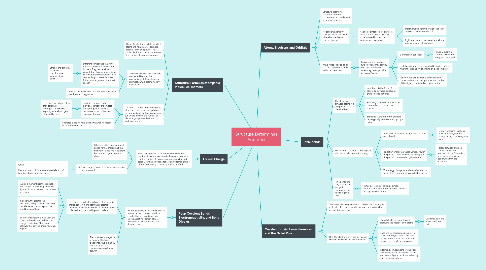# Structure Determines Properties

Get Started. It's FreeStructure Determines Properties## 1. Polar Covalent Bonds, Electronegativity, and Bond Dipoles

### 1.1. Electronegativity is the tendency of an atom to attract the electrons in a covalent bond toward itself. An electronegative element attracts electrons; an electropositive one donates them.

1.1.1. Electrons in covalent bonds are not necessarily shared equally. If one atom has a greater tendency to attract electrons the distribution is polarized, and the bond is polar covalent.

1.1.1.1. A dipole moment μ is the product of the amount of the charge multiplied by the distance between the centers of charge.

1.1.1.2. In general, the greater the electronegativity difference between two elements, the more polar the bond between them.

1.1.1.3. Electrostatic potential map, uses the colors of the rainbow to show the charge distribution. Blue (more positive) through red (more negative) regions

1.1.2. The most electronegative is fluorine, the least electronegative is lithium. Electronegativity decreases going down a column.

## 2. Formal Charge

### 2.1. Formal charges are the difference between the number of valence electrons in the neutral free atom and the number of valence electrons in its bonded state. Counting electrons for assigning formal charge differs from seeing if the octet rule is satisfied

2.1.1. When counting the electrons to assign formal charge, half the number of electrons in covalent bonds are assigned to each atom.

2.1.2. Formal charge = Group number in periodic table − Electron count

2.1.2.1. where Electron count = (Number of shared electrons) + Number of unshared electrons

## 3. Structural Formulas of Organic Molecules: Isomers

### 3.2. The order in which the atoms are connected is called the connectivity of the molecule and is almost always determined by experiment.

3.2.1. Different compounds that have the same molecular formula are isomers. They can be either constitutional isomers, sometimes called structural isomers (differ in connectivity) or stereoisomers (differ in arrangement of atoms in space).

3.2.1.1. Ethanol and dimethyl ether are constitutional isomers of each other.

3.2.2. Most of the time carbon has four bonds, nitrogen has three, and oxygen two.

### 3.3. Condensed formula: omit the bonds, atoms and their attached hydrogens are grouped and written in sequence; subscripts indicate the number of identical groups attached to a particular atom.

3.3.1. Bond-line formulas: labels for individual carbons are omitted and hydrogens attached to carbon are shown only when necessary for clarity.

3.3.1.1. Heteroatoms—atoms other than carbon or hydrogen—are shown explicitly as are hydrogens attached to them.

3.3.2. Unshared electron pairs are shown when necessary, but are often omitted.

## 4. Atoms, Electrons and Orbitals

### 4.2. Heisenber uncerainty principle: we only can tell where is more likely to find an electron.

4.2.1. Valence electrons: the outermost electrons, the ones most likely to be involved in chemical bonding and reactions.

4.2.1.1. Maximum number in the valence shell of any second-row element is 8.

4.2.1.2. Eight electrons in their valence shell is a complete octet of electrons.

### 4.3. Wave functions also called orbitals, tell where is likely to find and electron

4.3.1. Pauli exclusion principle: two electrons may occupy the same orbital only when they have opposite, or “paired,” spins

4.3.1.1. S orbitals are spherical.

4.3.1.1.1. Principal quantum number n: shell and energy of the orbital

4.3.1.2. Each 2p orbital, may be separated by nodal surfaces where the probability of finding an electron is zero.

4.3.1.3. Period of the periodic table of the element corresponds to the principal quantum number of the highest numbered occupied orbital

## 5. Ionic bonds

### 5.1. The attractive force between atoms in a compound is a chemical bond

5.1.1. Ionic bond, is the force of attraction between oppositely charged species (ions)

5.1.2. Positively charged ions are cations negatively charged ions are anions.

5.1.3. Elements at the left of the periodic table typically lose electrons, giving a cation.

### 5.2. The amount of energy to dislodge an electron is called ionization energy.

5.2.1. Processes that absorb energy are said to be endothermic.

5.2.1.1. Energy-releasing reactions are exothermic, and the energy change has a negative sign.

5.2.2. Ionization energy increases across a row in the periodic table. Elements at the right of the periodic table tend to gain electrons.

5.2.2.1. Forces between charged particles are called electrostatic, or Coulombic, and constitute an ionic bond when they are attractive.

5.2.3. The energy change for addition of an electron to an atom is referred to as its electron affinity

### 5.3. Ionic bonds are very common in inorganic compounds, but rare in organic ones.

5.3.1. Instead of losing or gaining electrons, carbon shares electrons with other elements making covalent bonds.

## 6. Covalent Bonds, Lewis Formulas, and the Octet Rule

### 6.2. Only the electrons in an atom’s valence shell are involved in covalent bonding

6.2.1. Shared electrons count toward satisfying the octet of both atoms

6.2.1.1. Unshared pairs are also called lone pairs.

6.2.2. Electrons are represented as dots in Lewis formulas, or Lewis structures. It usually represents bonds as lines and to sometimes omit electron pairs.

6.2.3. In forming compounds they gain, lose, or share electrons to achieve a stable electron configuration characterized by eight valence electrons.